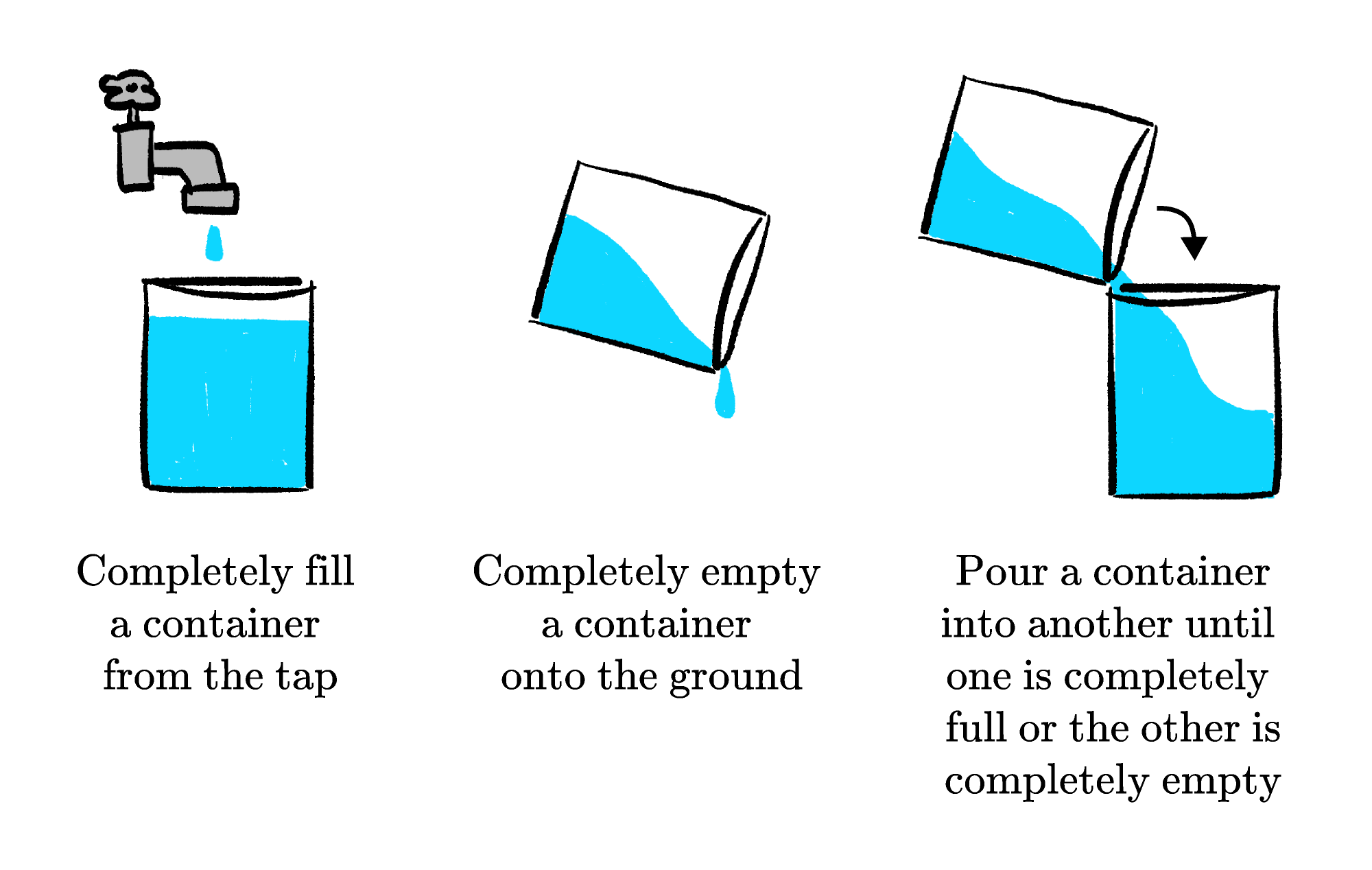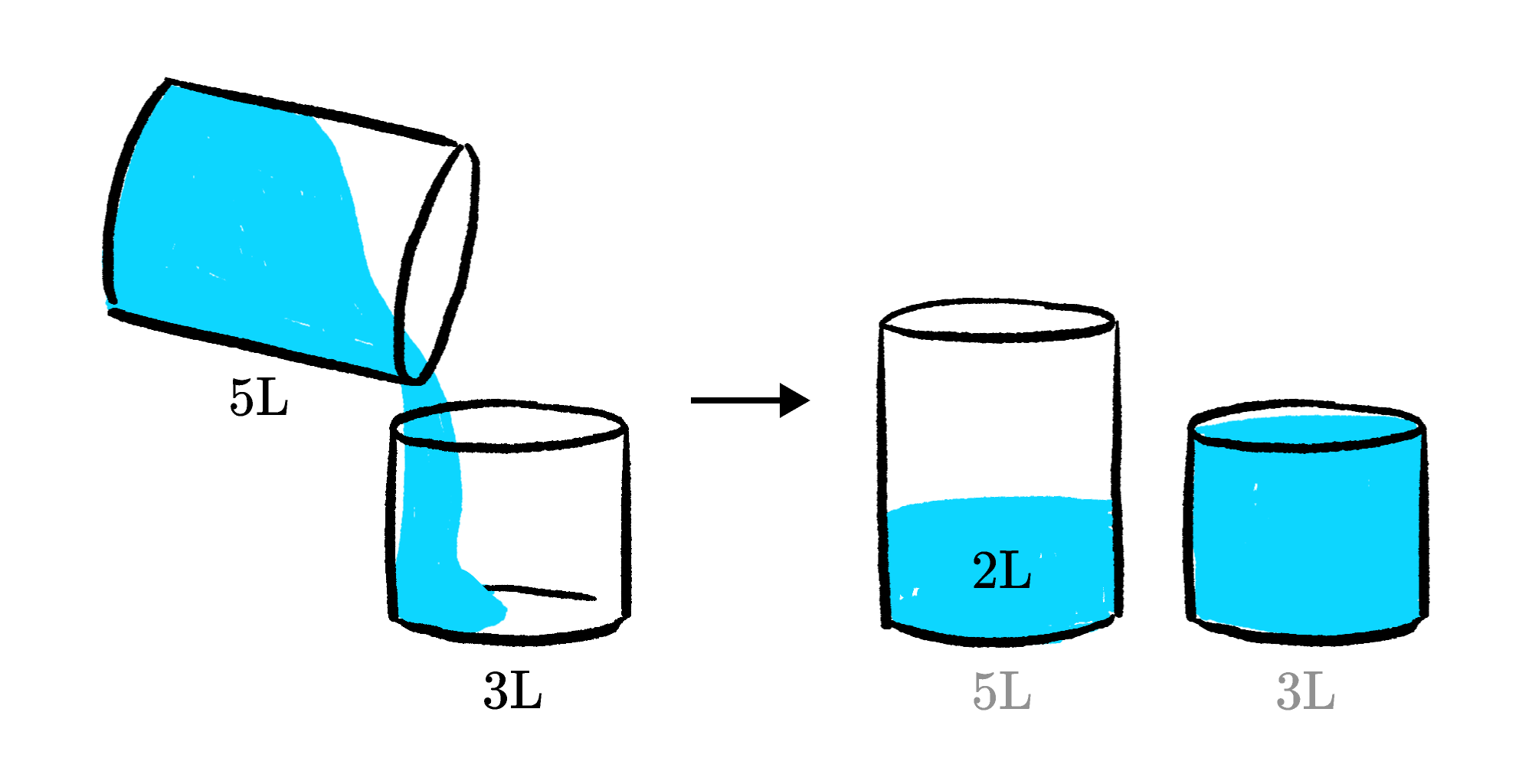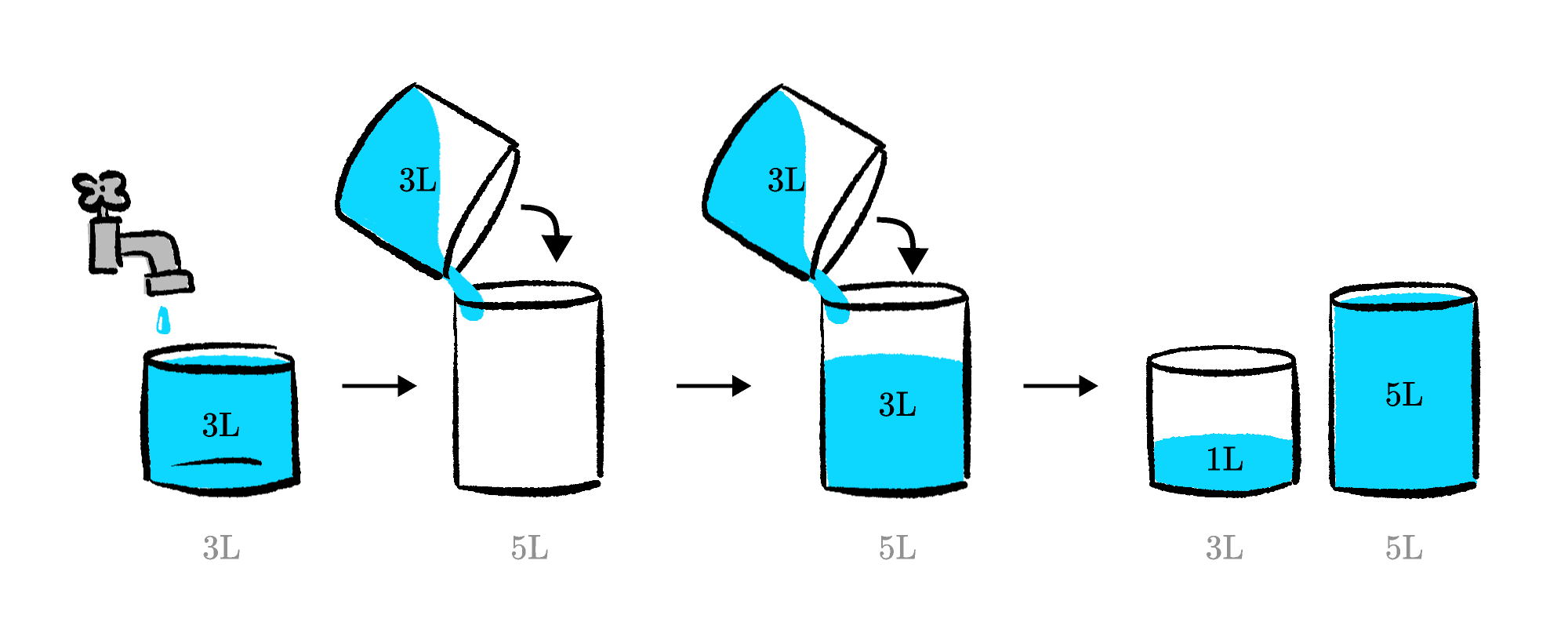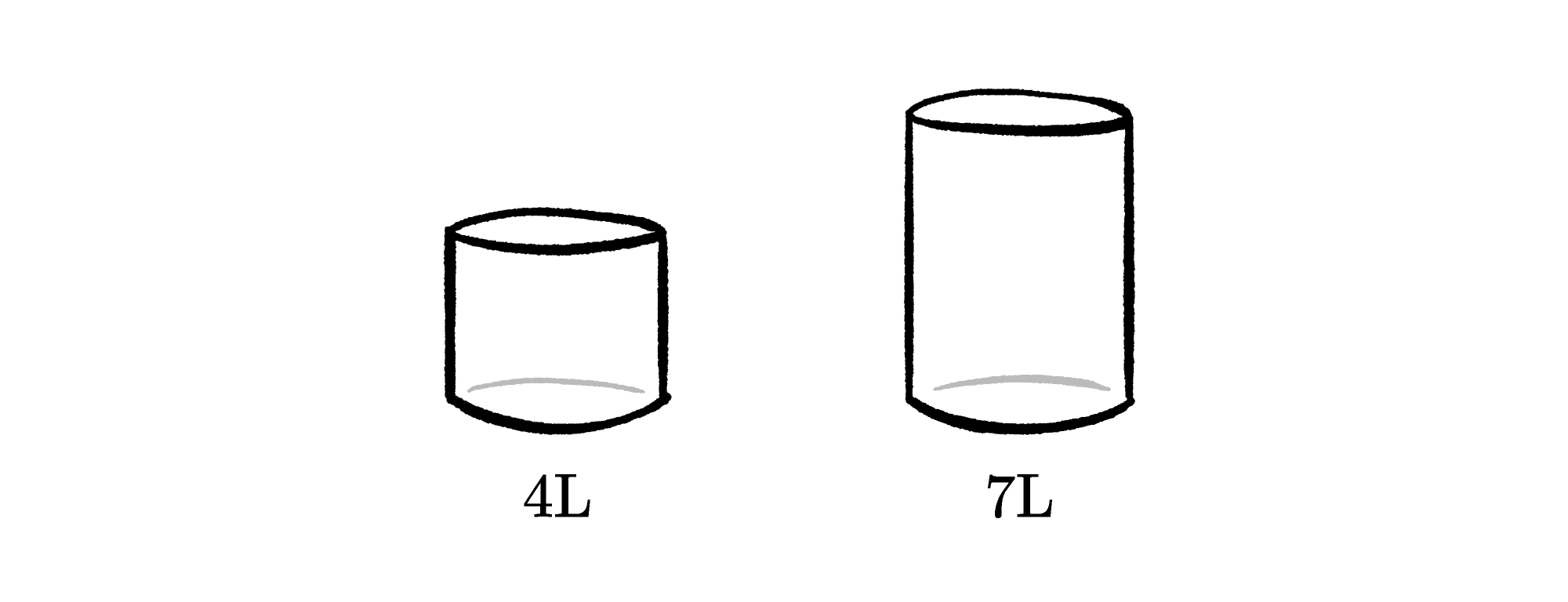Back

## Don't Waste Water!

Two containers: one holds $3$ liters and the other holds $5.$

The challenge: measure a specific quantity of liquid using only a sequence of actions chosen from the legal moves below.We can use these moves to measure amounts of water that are different from the exact measurements on the bottles. For example, if we fill the $5$-liter container and pour it into the $3$-liter container until it is full, we can be certain that we have $2$ liters remaining in the larger bottle.We can also combine multiple steps to find even more measurements. For example, we can measure out $1$ liter by doing the following:

1. Fill the $3$-liter bottle.
2. Empty the $3$-liter bottle into the $5$-liter bottle.
3. Refill the $3$-liter bottle.
4. Pour the $3$-liter bottle into the $5$-liter bottle until the larger bottle is full.

This leaves us with exactly $1$ liter left in the smaller bottle.# Today's Challenge

You have one container that holds $4$ liters and another container that holds $7$ liters.These are the only moves possible:

• Completely fill a container from the tap.
• Completely empty a container onto the ground.
• Pour a container into another until one is completely full or the other is completely empty.

Using only the moves listed above, what is the smallest amount of water you need to pour onto the ground in order to measure $2$ liters in either one of the containers?×